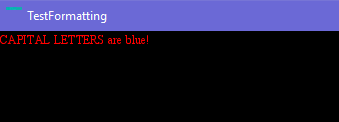# Implementing setTextFormat

I thought I’d attempt to implement the `setTextFormat` function in the `TextField` class but I’m having a bit of trouble understanding the TextEngine code base.

My current code is this:

``````import openfl.text.TextField;
import openfl.text.TextFormat;
import openfl._internal.text.TextFormatRange;

@:access(openfl._internal.text.TextEngine)
@:access(openfl._internal.text.TextFormatRange)
class ExtTextField extends TextField
{

public function new()
{
super();
}

override public function setTextFormat(format:TextFormat, beginIndex:Int = 0, endIndex:Int = 0):Void
{
var formatRange = new TextFormatRange(format, beginIndex, endIndex);
__textEngine.textFormatRanges.push(formatRange);

__layoutDirty = true;

__updateLayout();
}

}
``````

Now after looking at the code of the `__updateLayout()` I thought that in theory the format ranges would apply, but that didn’t seem to do anything. Without a debugger, it’s very difficult to identify exactly what the problem is.

The code I am testing with is the following:

``````        var txt = new ExtTextField();
txt.defaultTextFormat = new TextFormat("Times New Roman", 12, 0xFF0000);
txt.autoSize = TextFieldAutoSize.LEFT;
txt.selectable = false;
txt.text = "CAPITAL LETTERS are blue!";
txt.setTextFormat(new TextFormat("Times New Roman", 12, 0x0000FF), 0, 14);

``````

I have the following results with the above code:Not sure what I’m missing.

I managed it in the end:

``````if (__textEngine.textFormatRanges.length > 0) {
var range = __textEngine.textFormatRanges[__textEngine.textFormatRanges.length - 1];

range.end = beginIndex;

if (endIndex == 0)
endIndex = text.length;

var formatRange = new TextFormatRange(format, beginIndex, endIndex);
__textEngine.textFormatRanges.push(formatRange);
}
else
{
var formatRange = new TextFormatRange(format, beginIndex, endIndex);
__textEngine.textFormatRanges.push(formatRange);
}

__layoutDirty = true;

__updateLayout();

``````
1 Like

Hi @tienery, thanks for posting this! I’ve been pulling my hair over a ranged setTextFormat all day (works when targeting Flash, but not Mac), and this is the closest I’ve been to a solution so far.

I’m having some trouble getting this to work, though, and after many hours of struggling I thought I’d ask if you would mind sharing your final `ExtTextField` class or maybe explaining the second code example a bit more in-depth?

Ah, that only allows you to set formats linearly, meaning that you can only format a new range as long as the previously set range was before the new range, ie.

`setTextFormat(format, 16, 20);` only works after `setTextFormat(format, 0, 15);`

For a more robust solution, you can use my fork of OpenFL and use that as a `dev` repo in Haxelib using `haxelib dev` here.

Here is the code if you would prefer to use your own class:

``````if (endIndex > length || (endIndex == 0 && beginIndex > 0))
endIndex = length - 1;

if (beginIndex < 0)
beginIndex = 0;

if (__textEngine.textFormatRanges.length > 0)
{
var newFormatRanges = new Array<TextFormatRange>();
for (i in 0...__textEngine.textFormatRanges.length)
{
var range = __textEngine.textFormatRanges[i];
if ((beginIndex > range.end && endIndex > range.start) || (beginIndex < range.start && endIndex < range.end))
{
newFormatRanges.push(range);
continue;
}

var formatRange = new TextFormatRange(format, beginIndex, endIndex);
if (!(range.start < endIndex && range.end > beginIndex) || beginIndex == 0)
{
newFormatRanges.push(formatRange);
if (range.start < endIndex)
{
range.start = endIndex + 1 > length - 1 ? length - 1 : endIndex + 1;
}
else if (range.end > beginIndex)
{
range.end = beginIndex - 1 < 0 ? 0 : beginIndex - 1;
}
newFormatRanges.push(range);
}
else
{
if (range.start < endIndex)
{
range.start = endIndex + 1 > length - 1 ? length - 1 : endIndex + 1;
range.end = __textEngine.textFormatRanges[i + 1] != null ? __textEngine.textFormatRanges[i + 1].start - 1 : length;
}
var leftRange = new TextFormatRange(range.format, range.start, beginIndex - 1);
newFormatRanges.push(leftRange);
newFormatRanges.push(formatRange);
newFormatRanges.push(range);
}
}
__textEngine.textFormatRanges = newFormatRanges;
}
else
{
var formatRange = new TextFormatRange(format, beginIndex, endIndex);
__textEngine.textFormatRanges.push(formatRange);
}
__layoutDirty = true;
``````

This code needs to be in the `setTextFormat` function, and if you’re using your own class, you’ll need `@:access(openfl._internal.text.TextEngine)` there, I believe.

`TextFormatRange` is also in the same package, I believe.

There are plans to patch the pull request for this I made at some point, but of course that’s at the discretion of Josh.

1 Like

Works like a charm, thanks a lot!